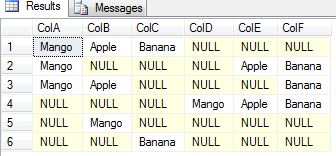# Count of total not null values from all columns of a table 2

In this post, we will count not null values from all the columns of a given table. One of my friend asked me to get the count of all not null values from all the columns of a given table. I used a CASE statement in my query and achieved this task but again i thought can i do this with the help of COUNT function too? And then i found an another way to achieve this task too. Here i am sharing both the ways. I am also sharing a trick to find only NULL values too.
To demonstrate this first create a table and put some demo data as below;

Create a Table:

```CREATE TABLE dbo.TEST
(
ColA VARCHAR(10),
ColB VARCHAR(10),
ColC VARCHAR(10),
ColD VARCHAR(10),
ColE VARCHAR(10),
ColF VARCHAR(10)
)
GO
```

```INSERT INTO dbo.TEST(ColA, ColB, ColC, ColD, ColE, ColF)
VALUES('Mango', 'Apple', 'Banana', NULL, NULL, NULL),
('Mango', NULL, NULL, NULL, 'Apple', 'Banana'),
('Mango', 'Apple', NULL, NULL, NULL, 'Banana'),
(NULL, NULL, NULL, 'Mango', 'Apple', 'Banana'),
(NULL, 'Mango', NULL, NULL, NULL, NULL),
(NULL, NULL, 'Banana', NULL, NULL, NULL)
```

Now this table has data like below;There are total 14 not null values in all the columns and 22 NULL values in all columns. And below we are trying to get this count in two different approaches.

## Approach 1 : Using CASE statement

To count NOT NULL values only

It’s so simple and the first one which comes in our mind;

```SELECT SUM(CASE WHEN ColA IS NULL THEN 0 ELSE 1 END
+ CASE WHEN ColB IS NULL THEN 0 ELSE 1 END
+ CASE WHEN ColC IS NULL THEN 0 ELSE 1 END
+ CASE WHEN ColD IS NULL THEN 0 ELSE 1 END
+ CASE WHEN ColE IS NULL THEN 0 ELSE 1 END
+ CASE WHEN ColF IS NULL THEN 0 ELSE 1 END) AS TotalNotNullCount
FROM dbo.TEST
```

#### Output:

14

To count NULL values only

In case you want to get the count of only NULL values, just reverse the logic inside CASE statement asCASE WHEN ColA IS NOT NULL THEN 0 ELSE 1 END.

## Approach 2 : Using COUNT Function

To count NOT NULL values only:

Hence COUNT function eliminates NULL values as any aggregate function do, we can also try this in a simple way to achieve this like below.

```SELECT COUNT(ColA) + COUNT(ColB) + COUNT(ColC) + COUNT(ColD) + COUNT(ColE) + COUNT(ColF) AS TotalNotNullCount FROM dbo.TEST
```

14

### To count NULL values only

In case you want to get the count of all NULL values only, you can try this COUNT(*) – COUNT(ColA) instead of COUNT(ColA) i.e. just subtract the count of total NOT NULL values from count of total values. COUNT(*) counts all rows even it has NULL in all the columns.
So what you suggest? It may be you also have some more techniques in your pocket and if it is, please don’t hesitate and just put your trick below in comment section, it may help someone by adding one more trick to achieve this task.

Rate This
[Total: 4   Average: 3.8/5]Gopal is a passionate Data Engineer and Data Analyst. He has implemented many end to end solutions using Big Data, Machine Learning, OLAP, OLTP, and cloud technologies. He loves to share his experience at https://www.sqlrelease.com/. Connect with Gopal on LinkedIn at https://www.linkedin.com/in/ergkranjan/.

This site uses Akismet to reduce spam. Learn how your comment data is processed.

## 2 thoughts on “Count of total not null values from all columns of a table”

•Milos

Is there any way to do this without this “walking” method mentioning all columns but to do it when you have for example 100 columns.

•Tim LaVenice

Great Post! I thought of one addition way to do this. You could divide the length of each field by itself. This would return a 1 for non-null values and null for null values. Then you could use the ISNULL function to convert the nulls to 0, and sum the results. To account for empty strings, you will need to add 1 to the length. Otherwise you’ll get 0 divided by 0, which results in a divide by zero error.

SELECT SUM(ISNULL((LEN(ColA)+1)/(LEN(ColA)+1),0) + ISNULL((LEN(ColB)+1)/(LEN(ColB)+1),0) + ISNULL((LEN(ColC)+1)/(LEN(ColC)+1),0) + ISNULL((LEN(ColD)+1)/(LEN(ColD)+1),0) + ISNULL((LEN(ColE)+1)/(LEN(ColE)+1),0) + ISNULL((LEN(ColF)+1)/(LEN(ColF)+1),0))
FROM dbo.TEST